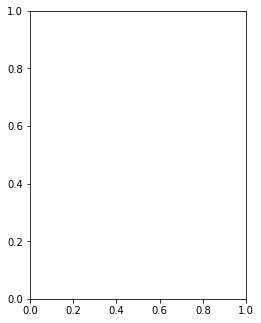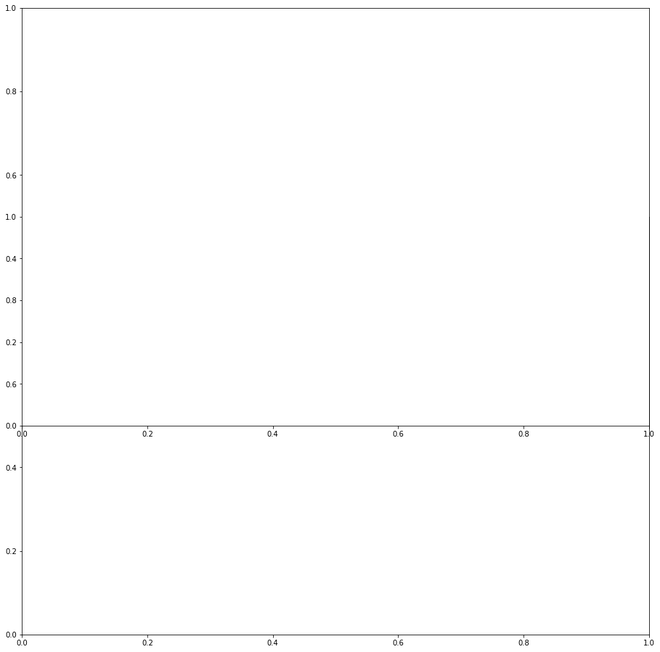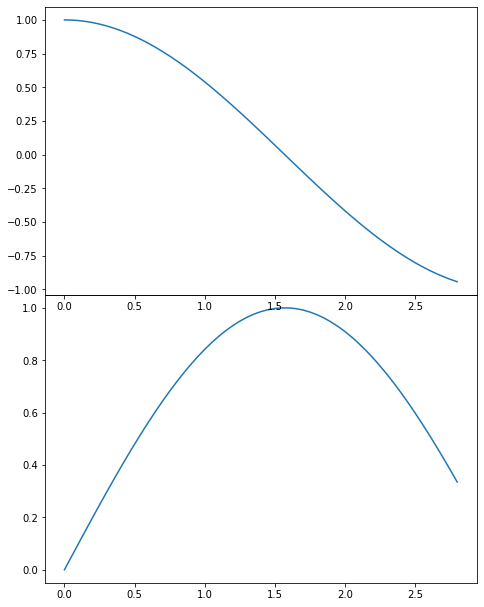Related Articles
How to Add Axes to a Figure in Matplotlib with Python?
• Difficulty Level : Easy
• Last Updated : 02 Dec, 2020

Matplotlib is a library in Python used to create figures and provide tools for customizing it. It allows plotting different types of data, geometrical figures. In this article, we will see how to add axes to a figure in matplotlib.

We can add axes to a figure in matplotlib by passing a list argument in the add_axes() method.

Parameters:

rect: This parameter is the dimensions [xmin, ymin, dx, dy] of the new axes. It takes the below elements as arguments in the list:

• xmin: Horizontal coordinate of the lower left corner.
• ymin: Vertical coordinate of the lower left corner.
• dx: Width of the subplot.
• dy: Height of the subplot.

Returns: This method return the axes class depends on the projection used.

Example 1:

## Python3

 `# Importing library``import` `matplotlib`` ` `# Create figure() objects``# This acts as a container``# for the different plots``fig ``=` `matplotlib.pyplot.figure()`` ` `# Creating axis``# add_axes([xmin,ymin,dx,dy])``axes ``=` `fig.add_axes([``0.5``, ``1``, ``0.5``, ``1``])`` ` `# Depict illustration``fig.show()`

Output:Example 2:

## Python3

 `# Importing library``import` `matplotlib`` ` `# Create figure() objects``# This acts as a container ``# for the different plots``fig``=``matplotlib.pyplot.figure() `` ` `# Creating two axes``# add_axes([xmin,ymin,dx,dy])``axes``=``fig.add_axes([``0``,``0``,``2``,``2``]) ``axes1``=``fig.add_axes([``0``,``1``,``2``,``2``])`` ` `# Depict illustration``fig.show()`

Output:Example 3:

## Python3

 `# Import libraries``import` `matplotlib``import` `numpy`` ` `# Create figure() objects``# This acts as a container``# for the different plots``fig ``=` `matplotlib.pyplot.figure()`` ` `# Generate line graph``x ``=` `numpy.arange(``0``, ``1.414``*``2``, ``0.05``)``y1 ``=` `numpy.sin(x)``y2 ``=` `numpy.cos(x)`` ` `# Creating two axes``# add_axes([xmin,ymin,dx,dy])``axes1 ``=` `fig.add_axes([``0``, ``0``, ``1``, ``1``])``axes1.plot(x, y1)``axes2 ``=` `fig.add_axes([``0``, ``1``, ``1``, ``1``])``axes2.plot(x, y2)`` ` `# Show plot``plt.show()`

Output:Attention geek! Strengthen your foundations with the Python Programming Foundation Course and learn the basics.

To begin with, your interview preparations Enhance your Data Structures concepts with the Python DS Course. And to begin with your Machine Learning Journey, join the Machine Learning – Basic Level Course

My Personal Notes arrow_drop_up